[TOC]

# 1. Introduction to Maxflow

### Min-cut pb

• input: edge-weighted digraph G, each edge `e` has weight("capacity") `c[e]`>=0, a source vertex `s`, a target vertex `t`.
• def. an st-cut `(A,B)` is a partition of vertices into 2 disjoint sets A and B, with `s` in set `A` and `t` in set `B`.
• def. the capacity of a cut `(A,B)` is sum of capacities of edges going from A to B (not considering B to A).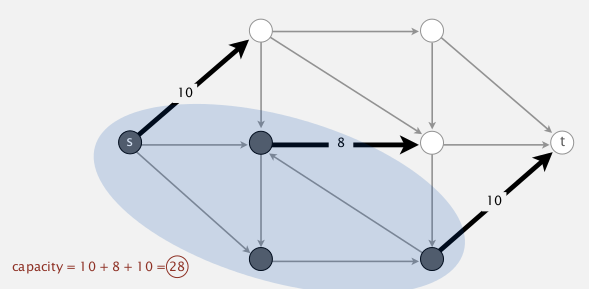⇒ min-cut pb: find the cut (A,B) with min-capacity.

### Max-flow pb

• same input: graph G, source s, target t
• def. an st-flow is an assignment of values to edges `f: e→f[e]` such that:
• capacity constraint: `0<=f[e]<=c[e]` for any e;
• local equilibrium: for any vertex v (other than s or t), inflow=outflow;
• def. the value of a flow `f` is the inflow at `t`. (assume no ingoing edge to s or outgoing edge to t)⇒ max-flow pb: find `f` with max value.

remark: max-flow and min-cut are dual problems.

# 2. Ford-Fulkerson Algorithm

def. given a flow `f` for a graph, an "augment path" is an undirected path form `s` to `t`, if there exist `df>0` ("bottleneck capacity") such that:

• for forward edges e: can augment flow by `df` (not full: `f[e]+df<=c[e]`)
• for backward edges: can decrease flow by `df` (not empty: `f[e]-df>=0`)• def. residual capacity
• for forward edge e, residual-cap = c[e]-f[e]
• for backward edge e, residual-cap = f[e]

⇒ an aug-path is a path where each edge has residual capacity >0.

blocking edges: full forward edge or empty backward edge.

→ idea: increase flow along augment paths.
[algo]

• start: 0 flow: `f[e]=0` for all e.
• find an augment path (and the corresponding `df`) in graph, and change the flows along the path by `+/-df`.
• loop until no augment path exists. (ie. all path s→t are blocked either by a full forward edge or an empty backward edge, ie. by an edge with 0 residual capacity)FF is a gernel algorithm:# 3. Maxflow-Mincut Theorem

def. for a cut (A,B), the net flow across the cut (netflow(A,B)) is the sum of flows from A to B minus flows from B to A.

[flow-value Lemma]
For any flow `f` and any cut `(A,B)`netflow(A,B) = value(f).
pf.
induction on the size of set B.
base case, when B={t}, by def we have netflow(A,B) = value(f)

when moving any vertex v from A to B:
* netflow(A, B) augment by flow(A→v)+flow(B→v)=inflow(v),
* netflow(A, B) decrease by flow(v→A)+flow(v→B)=outflow(v),
* by equilibrium of flow, netflow(A',B')=netflow(A,B)=value(f)

ex. (A: gray vertices, B: white vertices)[cor] outflow(s)=inflow(t)=value(f)

[weak duality]
For any flow `f` and any cut `(A,B)`, ⇒ value(f) <= capacity(A,B).

[Augmenting path Th]
A flow `f` is maxflow iff there is no augment path.

[maxflow-mincut Th]
value(maxflow) = capacity(mincut).

pf.
for any flow `f`, prove the equivalence of the 3 following statements:
i. there exists a cut st: capacity(cut) = value(f).
ii. `f` is a maxflow.
iii. there is no augmenting path wrt `f`.

• [i⇒ii]

suppose cut(A,B) st: capacity(A,B)=value(f)
⇒ by weak duality, for any other flow f', vlaue(f')<=capacity(A,B)=value(f)

• [ii⇒iii] (eqv to prove ~iii⇒~ii)

suppose there is an aug-path from s to t, of bottleneck capacity=df,
⇒ by improving f with df, we get a f' > f

• [iii⇒i]

suppose there is no aug-path, ie, all path from s to t are blocked by some full-forward edge or empty backward edge.
⇒ let A:=vertices connected with s by a path with no blocking edges, and B := the rest
(so once we get a maxflow, we can compute the mincut in this way)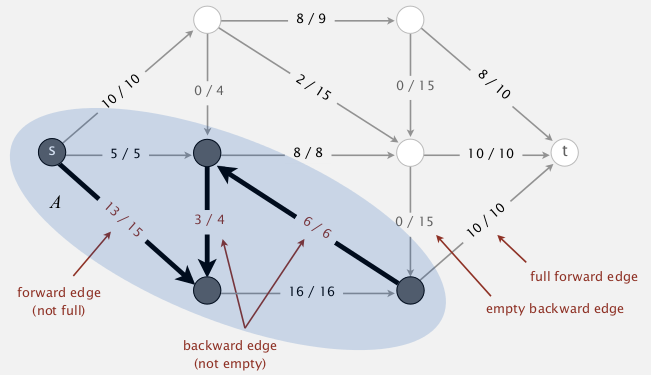→ for all edges across A and B, all forward edges are full, all backward edges are empty
⇒ capacity(A,B) = netflow(A,B) = value(f) by flow-value lemma
CQFD... 过瘾...

# 4. Running Time Analysis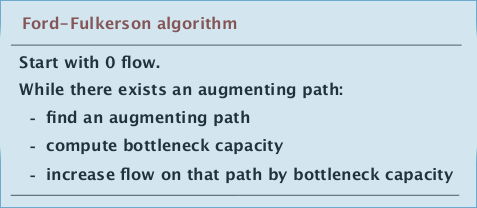• getting a mincut form maxflow? → easy (as discussed in the pf above)
• computing an aug-path? → BFS
• does FF algo always terminate? how many augmentations? → ...

### integer capacity graphs

special case of FF algo: edge capacities are integers between 1 and U.

invariant: flow is always integer all along FF algo.

[prop] nb of augmentations <= value of maxflow.
pf. each augmentation will add flow by >=1.

[integrality Th] There exist an integer-valued maxflow.

### Bad case for FF

nb of augmentation == value of maxflow
(each time, the path through the middle edge is chosen as aug-path)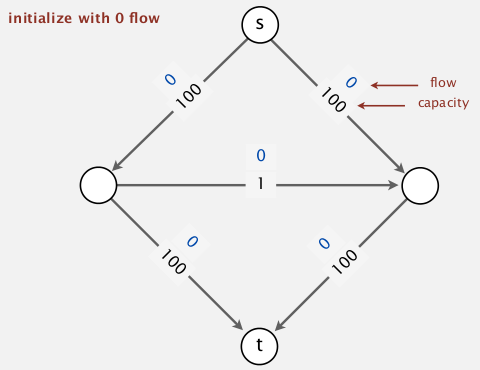can be easily avoided⇒ by using shortest(nb of edges)/fastest(biggest df) path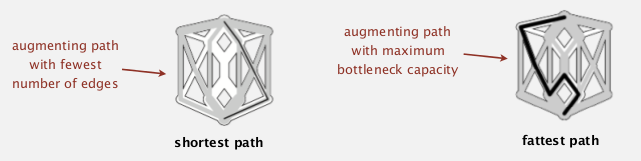Performance of FF depends on the algo for choosing aug-path: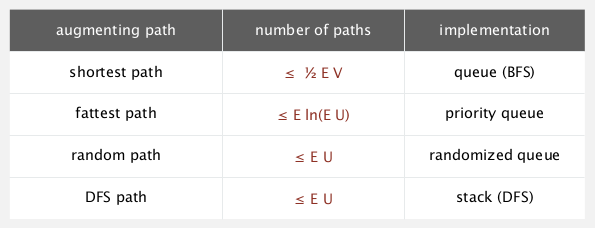# 5. Java Implementation

### representation of flow graph

• flow edge:

each e= v→w, have flow f[e] and capacity c[e].

• flow graph:

put e in both v and w's adj-list.

• flow augmentation (by delta)
• for forward edge e, f[e] += delta
• for backward edge e, f[e] -= delta

### Residual graph Gr

def. For a flow `f` and a graph `G`, the residual graph `Gr` is obtained by:

for each edge `e=v→w`, (with `c[e]` and `f[e]`) in `G`, put in `Gr`:
`e1=v→w`, with weight=`c[e]-f[e]`

`e2=w→v`, with weight=`f[e]` (即两个方向上的weight都为residual capacity)

(rmq: `Gr` is just a weighted digraph, not a flow graph)

[prop] Augment path in `G` is equivalent to a path in `Gr` (`df` of aug-path in `G` = min edge weight in `Gr`).
(但是实现的时候其实不用显式构造Gr, 只需BFS的时候修改一下即可)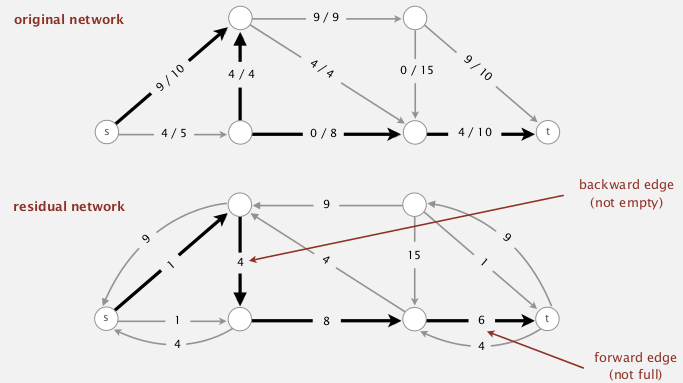### APIs

• flow-edge:
rmq. both calculate residual-cap and augmentation need to specify a direction, so we need a index v as parameter for these 2 functions.

```public class FlowEdge{
private final int v, w;
private final double capacity;
private double flow=0.0;
FlowEdge(int v, int w, double cap);
int from();
int to();
int other(int v);
double capacity();
double flow();
double residualCapTo(int v);// residual capacity
void addFlowTo(int v, double delta);// augment residual flow
}
```
• flow graph:

```public class FlowNetwork{
private Bag<FlowEdge>[] adj;//use adj-list representation for flow graph
FlowNetwork(int V);
void addEdge(FlowEdge e);
Iterable<FlowEdge> adj(int v);// both incoming and outgoing edges
...
}
```
• FF algo:

• use a function `hasAugPath()` to test termination
• use a function `bottleNeck()` to get delta
• if a augpath is found, use two arrays `reached[]` and `edgeTo[]` to get the augpath (find the path backwards).

code:

```public class FordFulkerson{
private boolean[] reached; //reached[v] indicates if a path s-->v exists in Gr, used in DFS
private FlowEdge[] edgeTo;// edgeTo[v] = last edge on the path s-->v
private double value=0.0;// value of flow
public FordFulkerson(FlowNetwork G, int s, int t){
while(this.hasAugPath(G,s,t)){
double delta = this.bottleNeck();
for(int v=t; v!=s; v=edgeTo[v].other(v))
edgeTo[v].addFlowTo(v, delta);
this.value += delta;// each time the flow value augments by delta
}
}
private double bottleNeck(){//bottleneck-cap = min residual flow on the aut-path
double bottleneck = 9999999;
assert(reached[t]);// the aug-path should exsit
for(int v=t; v!=s; v = edgeTo[v].other(v))
bottleneck = Math.min(bottleneck, edgeTo[v].);
return bottleneck;
}
private boolean hasAugPath(FlowNetwork G, int s, int t){
// perform a BFS
Queue<Integer> q = new LinkedList<Integer>();
this.reached = new boolean[G.V()];
this.edgeTo = new FlowEdge[G.V()];
q.add(s);
while(!q.isEmpty()){
int v = q.deque();
for(FlowEdge e:G.adj(v)){
int w = e.other(v);
if(!reached[w] && e.residualCapTo(w)>0){// modified BFS: valid edges are those with  residualCap>0
edgeTo[w] = e;
reached[w] = true;
if(w==t) return true;// t is reached by BFS
q.enqueue(w);
}
}
}// BFS while loop
return false;
}
}//class FF
```

# 6. Maxflow Applications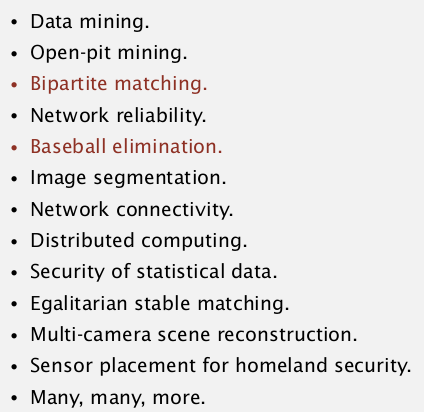### ex1. bipartite matching pb⇒ is there a way to match all students to a job?
ie. given a bipartite graph, find a perfect matching.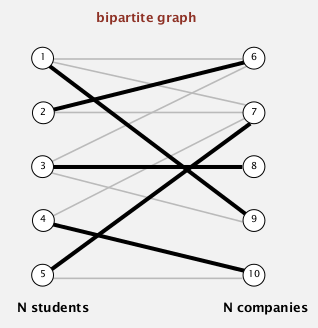modeling

• add source `s` and target `t`
• all edges from `s` to students: capacity=1
• all edges from companies to `t`: capacity=1
• all edges from student to company: capacity=INF

⇒ find maxflow in the graph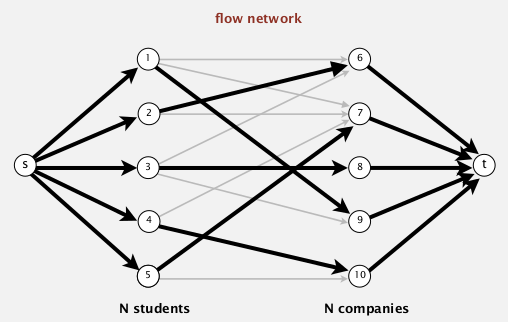when no perfect matching: mincut can explain why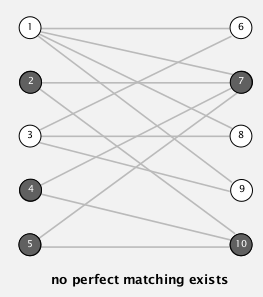in the above case, student 2,4,5 can only be matched to 7,10
⇒ mincut can help us find such cases!

recall: how to get mincut from maxflow

mincut = (A,B), where:
A:=vertices connected with s by a path with non blocking edges,
B := the rest
(blocking edges: full forward edge or empty backward edge on path)

ex.• let S=students on s side of mincut (in above case, S={2,4,5})
• let T=companies on s side of mincut (in above case, T={7,10})
• |S|>|T|, that's why no perfect matching!

### ex2. baseball elimination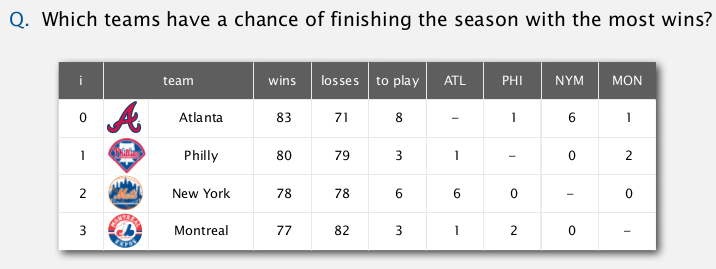(前三列是目前成绩, 后面四列是接下来赛程矩阵)
Montreal is mathematically eliminated → easy to see
→ Philly is mathematically eliminated also !

• another case: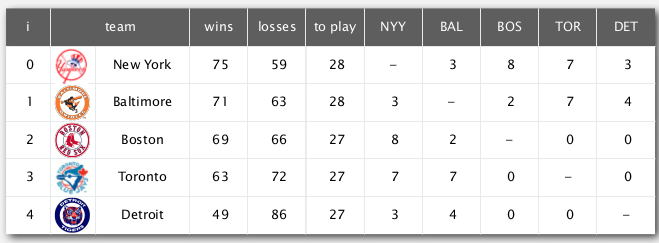Detroit is mathematically eliminated !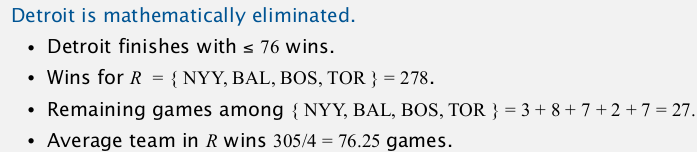whether team-4 still has a chance to win?
modelling

• remaining games flow from s to t.
• use team-pairs ans teams as vertices
• carefully chosen capacities(see below)⇒ team 4 could win iff all flow from s are full (ie. all match points can be repartitioned over other teams without depassing team 4's maximum wins).

#### Part 5 of series «Algorithms Princeton MOOC II»：

comments powered by Disqus